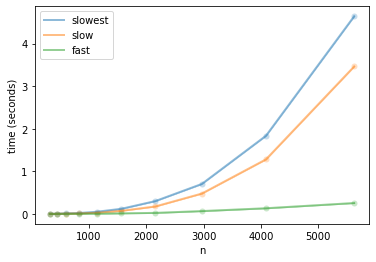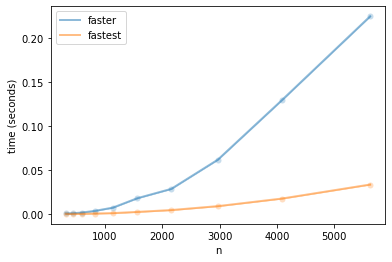# Fast rank-one updates to matrix inverse?

Let $A \in \mathbb{R}^{n \times n}$. The Sherman–Morrison formula suggests a computational shortcut to update a matrix inverse subject to a rank-one update, i.e., an additive change of the form $A + u \, v^\top$ where $u, v \in \mathbb{R}^n$:

$$(A + u \, v^\top)^{-1}$$

Suppose we have precomputed $B = A^{-1}$, the shortcut is $$(A + u \, v^\top)^{-1} = B - \frac{1}{1 + v^\top B\, u} B\, u\, v^\top B$$

Implemented carefully, it runs in $\mathcal{O}(n^2)$, which is better than $\mathcal{O}(n^3)$ from scratch. Ok, technically matrix inversion can run faster than cubic, but you get my point.

Below is a direct translation of equations into numpy:

B -= B @ u @ v.T @ B / (1 + v.T @ B @ u)


However, it does NOT run in $\mathcal{O}(n^2)$. Do you see why?

In :
import numpy as np, scipy
from numpy.linalg import inv

def slowest(A,B,u,c):
return inv(A + np.outer(u, v))

def slow(A,B,u,v):
return B - B @ u @ v.T @ B / (1 + v.T @ B @ u)

def fast(A,B,u,v):
return B - (B @ u) @ (v.T @ B) / (1 + v.T @ B @ u)

In :
from arsenal import iterview, timers
for n in iterview(np.logspace(2.5, 3.75, 10)):
n = int(np.ceil(n))
A = np.random.randn(n,n)
B = inv(A)
u = np.random.randn(n,1)
v = np.random.randn(n,1)
yield (n, A, B, u, v)

In :
T = timers()
for (n, A, B, u, v) in workload():
with T['slowest'](n=n):
x = slowest(A,B,u,v)
with T['slow'](n=n):
y = slow(A,B,u,v)
with T['fast'](n=n):
z = fast(A,B,u,v)
# pro tip: always check correctness when optimizing your code!
assert np.allclose(x, y)
assert np.allclose(x, z)

100.0% (10/10) [======================================================] 00:00:24

In :
T.plot_feature('n');In :
T.compare()

fast is 10.8188x faster than slow (p=0.08099, mean: slow: 0.552587, fast: 0.0510765)
fast is 15.0779x faster than slowest (p=0.03201, mean: slowest: 0.770126, fast: 0.0510765)


What's going on?

As you can see in the plots, the slow and slowest algorithms run at similar speeds despite all that extra effort to implement the fancy formula. As expected, when carefully implemented, the rank-one update method is lightning fast.

I suspect that this mistake is quite common. By default, multiplication is a left-associative operation. This case results in an unnecessary matrix-matrix product instead of an outer-product of two matrix-vector products.

Looking forward, I suggest that authors put explicit parenthesis in the rank-one update formula:

$$(A + u \, v^\top)^{-1} = B - \frac{1}{1 + v^\top B\, u} \left(B\, u\right)\, \left(v^\top B\right)$$

### Bonus 🔥¶

To make this method really fast, we can use specialized BLAS routines for rank-one updates. Thanks to Dr. Nikos Karampatziakis for suggesting it on Twitter.

Specifically, DGER, which computes $$B \gets B + \alpha \, x\, y^\top$$

The following methods have a slightly different API as they do updates to $B$ in place.

In :
def fastest(B,u,v):
# Warning: overwrite_a=True silently fails when B is not an order=F array!
assert B.flags['F_CONTIGUOUS']
Bu = B @ u
alpha = -1 / (1 + v.T @ Bu)
scipy.linalg.blas.dger(alpha, Bu, v.T @ B, a=B, overwrite_a=1)

In :
def faster(B,u,v):
Bu = B @ u
np.subtract(B, Bu @ (v.T @ B) / (1 + v.T @ Bu), out=B)

In :
T2 = timers()
for (n, A, B, u, v) in workload():
ref = fast(A, B, u, v)

x = B.copy()
with T2['faster'](n=n):
faster(x,u,v)
assert np.allclose(ref, x)

y = np.array(B.copy(), order='F', copy=True)
with T2['fastest'](n=n):
fastest(y,u,v)
assert np.allclose(ref, y)

100.0% (10/10) [======================================================] 00:00:12

In :
T2.plot_feature('n');In :
T2.compare()

fastest is 6.8440x faster than faster (p=0.03783, mean: faster: 0.0477374, fastest: 0.0069751)


## Closing¶

Be careful translating math into code. Also, if you have a symmetric matrix, such as a covariance or Kernel matrix, it is generally more efficient (in terms of constant factors) and numerically stable to perform rank-one updates to the Cholesky factorization.

Acknowledgements: I'd like to thank Nikos Karampatziakis and Suzanna Sia for comments, corrections, and/or suggestions that improved this article.